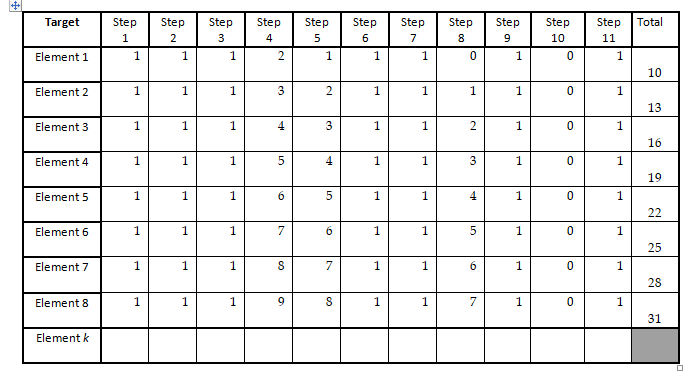Home / Expert Answers / Computer Science / as-you-will-see-in-chapter-3-one-measure-of-work-done-by-an-algorithm-is-the-number-of-compariso-pa158

# (Solved): As you will see in Chapter 3, one measure of work done by an algorithm is the number of compariso ...

As you will see in Chapter 3, one measure of work done by an algorithm is the number of comparisons of elements that the algorithm must perform. In the Search for Value algorithm, the comparisons are made in Step 5. Under the assumption that the target is as likely to be in any one position in the list as in any other position, the best way to measure the number of comparisons is to average the number of comparisons required for the various positions of the target. Compute the average number of times that Step 5 must be executed for the eight-element list by averaging the numbers in the first eight rows of the Step 5 column of the table in Exercise 2.4. If the list only had seven elements, we would average the first seven of the numbers in this column. Try computing this average for a few values for the list size. Give a formula for the average number of comparisons if there are n elements in the list.We have an Answer from Expert

To compute the average number of times that step 5 must be executed for list of n elements using search for value algorithm, you would need to average the numbers in the step 5 column of the table for first n rows.

The formula for calculating average is

Average of Step 5 executions =

This formula represents sum of integers from 1 to n divided by n.

In this case, list has 8 elements, the average of step 5 executions would be

Therefore, Step 5 of the search for value algorithm would need to be executed 4.5 times for 8-element list.
We have an Answer from Expert